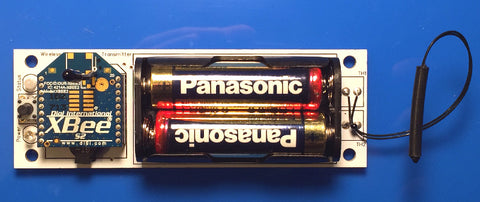# Thermistors, PICs, Steinhart-Hart and Wireless Applications

One of the most basic sensors in many wireless applications is a temperature sensor. The thermistor is widely used for its low cost, it's easy to use and has good accuracy. Here is a dual temperature sensor (VP-TX-190) that uses thermistors, is wireless (Zigbee) and uses the Steinhart-Hart equation for the temperature value.

VP-TX-190 front view:VP-TX-190 Rear View:In this circuit, the processor used is a PIC18LF14K22 and the thermistor input circuit is:Simple, right? The +V can be supplied from one of the processor pins, enabling the wireless application to have very low power drain during sleep modes. In this example, the thermistor used is a 10K type with a Beta value of 3380. (There are many good articles available on the web explaining the theory behind thermistors, Beta values, etc.) R2, C1 and R3 is a high pass filter to help eliminate high frequency noise.

In all of our circuits that use thermistors, we use the Steinhart-Hart equation to get the actual temperature value in Degrees C. By using this equation, the +V supply can be any value, the AD counts are based on the reference input to the AD converter, in our case, the supply voltage to the PIC is 3.3 VDC and the V+ (whether from the power supply or PIC pin) is also 3.3 VDC

This code excerpt (we write all our application code for PIC based products in C) shows how the simple circuit above coupled with the Steinhart-Hart equation gives a temperature value:

`// Code based on PIC18LF14K22 Processor// V+ turned on by the PIC pin C3// Channel 3 used for temperature input THv#include <math.h>#define R_LOAD              10000.0#define R_ROOM_TEMP         10000.0      /* For 25 Deg C  */#define T_BETA              3380.0#define T_AD_COUNTS         1024.0       /* 10 bit AD */ #define ROOM_TEMP_NOM_T1    25.0         /* For 25 Deg C */int Update_Temperature(void){    int i;    unsigned int average;    float sample;    TRISCbits.TRISC3 = 0;       /* Define Pin C3 As Output */    LATCbits.LATC3 = 1;         /* Turn ON Thermistor*/    TRISAbits.TRISA4 = 1;    ANSELbits.ANSEL3 = 1;       ADCON2 = 0b10000000;    ADCON1 = 0b00000000;    ADCON0 = 0b00001101;        /* Select Channel 3*/    average = 0;    ConvertADC();    while(BusyADC());    average = ReadADC();    LATCbits.LATC3 = 0;         /* Turn OFF Thermistor*/    /* Here is the Steinhart-Hart temperature calculation */    sample = (T_AD_COUNTS / average) -1;    sample = R_LOAD / sample;    sample = sample / R_ROOM_TEMP;    sample = log(sample);    sample /= T_BETA;    sample += 1.0 / (ROOM_TEMP_NOM_T1 + 273.15);    sample = 1.0 / sample;    sample -= 273.15;    return (int)(sample);}int ReadADC(void){    return (((unsigned int)ADRESH)<<8)|(ADRESL);}void ConvertADC(void){    ADCON0bits.GO = 1;}char BusyADC(void){    return(ADCON0bits.GO);}`

Save